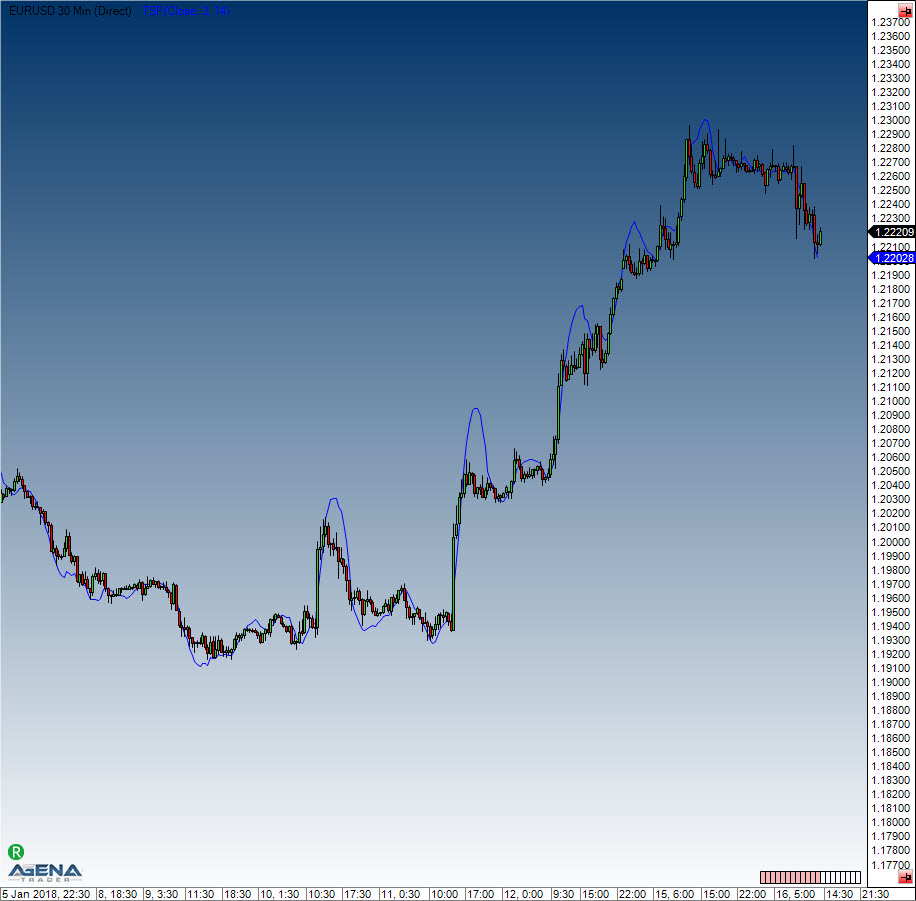# Time-Series-Forecast (TSF)

#### Description

The Time-Series-Forecast is quite similar to a moving average. Here, the trend is established based on a regression equation that uses the smallest square formula. The goal of the TSF is to anticipate future price movements with the help of currently existing data.

#### Interpretation

The TSF reacts faster than the moving averages. These always have time delays when depicting the pre-existing trends. The TSF formula never allows the distance to the current price to become too large, thus permitting sharper trade reversal recognition.
An entry is placed when the price breaks the TSF line from bottom to top.

#### Usage

TSF(int forecast, int period)
TSF(IDataSeries inSeries, int forecast, int period)
TSF(int forecast, int period)[int barsAgo]
TSF(IDataSeries inSeries, int forecast, int period)[int barsAgo]

#### Return value

double
When using this method with an index (e.g. TSF(3,14)[int barsAgo] ), the value of the indicator will be issued for the referenced bar.

#### Parameters

forecast Number of bars used for the forecast
inSeries Input data series for the indicator
period Number of bars included in the calculations

#### VisualizationTime-Series-Forecast (TSF)

#### Example

//Output the TSF values
Print("The current value for the TSF is: " + TSF(3, 14));